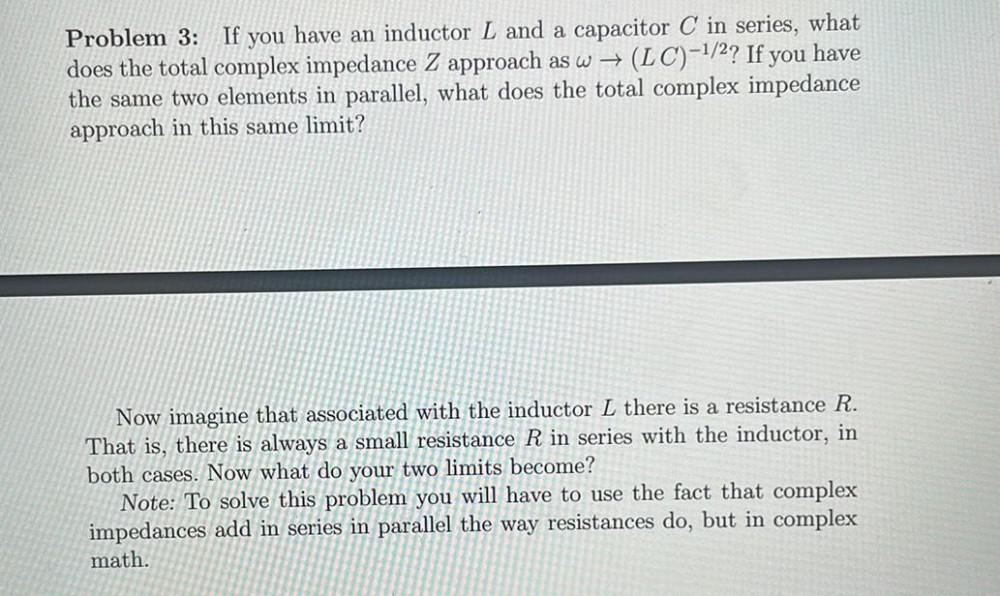Question:

# Problem 3: If you have an inductor L and a capacitor C in series, what does the total complex impedance Z approach as w → (LC)-1Problem 3: If you have an inductor L and a capacitor C in series, what does the total complex impedance Z approach as w → (LC)-1/2? If you have the same two elements in parallel, what does the total complex impedance approach in this same limit? Now imagine that associated with the inductor L there is a resistance R. That is, there is always a small resistance R in series with the inductor, in both cases. Now what do your two limits become? Note: To solve this problem you will have to use the fact that complex impedances add in series in parallel the way resistances do, but in complex math.